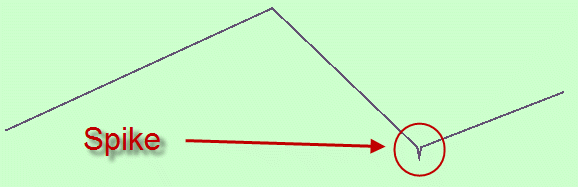# Removing Spikes in SQL Server Spatial Linestrings

## Introduction

The creation of spatial data from GPS observations is a universal thing for GIS today.

Linestring data can be created from a sequence of GPS point observations or GPS tracks.

In either case, it is common to see spikes within the linestrings formed from GPS data.

A spike is “a sharp, abrupt and narrow deviation from the normal course of a linestring”.

Graphically it can look like this:Or this:

Or this:

## Removing Spikes

The method I take for removing or resolving spikes is to systematically take three sequential vertices and compute the deflection angle subtended in the middle. If the subtended angle is less than some threshold value, a spike exists and is resolved by removing the middle vertex. The next three vertex triplet can then be selected and processed (formed from the remaining 2 vertices plus the next one) until finished.

### Issue

There is an issue that must be raised. That is that if a linestring is invalid (STIsValid() = 0) – see second example above – vertex access functions such as STNumPoints() and STPointN() do not work.

```with data as (
select geometry::STGeomFromText('LINESTRING(-1 1,0 0,0 1,0 0,1 1)',0) as geom  -- Invalid linestring see second example above.
)
select a.geom.STNumPoints() as numPoints, a.geom.STPointN(2) as point
from data as a;

Msg 6522, Level 16, State 1, Line 4
A .NET Framework error occurred during execution of user-defined routine or aggregate "geometry":
System.ArgumentException: 24144: This operation cannot be completed because the instance is not valid. Use MakeValid to convert the instance to a valid instance. Note that MakeValid may cause the points of a geometry instance to shift slightly.
System.ArgumentException:
at Microsoft.SqlServer.Types.SqlGeometry.ThrowIfInvalid()
at Microsoft.SqlServer.Types.SqlGeometry.STNumPoints()
```

### Functions

So that a common function can be used to process all three examples above, the best method is to have the function process Well Known Text (WKT).

Here is such a function:

```CREATE FUNCTION [dbo].[STRemoveSpikesByWKT]
(
@p_linestring      varchar(max),
@p_srid            int   = 0,
@p_angle_threshold float = 0.5,
@p_round_xy        int   = 3,
@p_round_z         int   = 2,
@p_round_m         int   = 2
)
Returns varchar(max)
AS
....

-- With a wrapper function that calls it.

CREATE Function [dbo].[STRemoveSpikes]
(
@p_linestring      geometry,
@p_angle_threshold float = 0.5,
@p_round_xy        int   = 3,
@p_round_z         int   = 2,
@p_round_m         int   = 2
)
Returns geometry
AS
...
```

### Examples

Some examples as provided below which process two of the examples shown in the introduction.

```with data as (
select geometry::STGeomFromText('LINESTRING(-1 1,0 0,0 1,0 0,1 1)',0) as geom
), spikeRemoval as (
select geometry::STGeomFromText([dbo].[STRemoveSpikesByWKT](a.geom.STAsText(),a.geom.STSrid,1.0,3,2,1),a.geom.STSrid) as geom
from data as a
)
select a.geom.STIsValid() as isValid, a.geom.STAsText() as geom
from spikeRemoval as a;

isValid geom
1       LINESTRING (-1 1, 0 0, 1 1)
```
```with data as (
select geometry::STGeomFromText('LINESTRING(-1 1,-0.025 0,0 1,0.025 0,1 1)',0) as geom
), spikeRemoval as (
select [dbo].[STRemoveSpikes](a.geom,5.0,3,2,1) as geom
from data as a
)
select a.geom.STIsValid() as isValid, a.geom.STAsText() as geom
from spikeRemoval as a;

isValid geom
1       LINESTRING (-1 1, -0.025 0, 0.025 0, 1 1)
```

These functions are available in the collection of TSQL functions available from the Shop on this website.